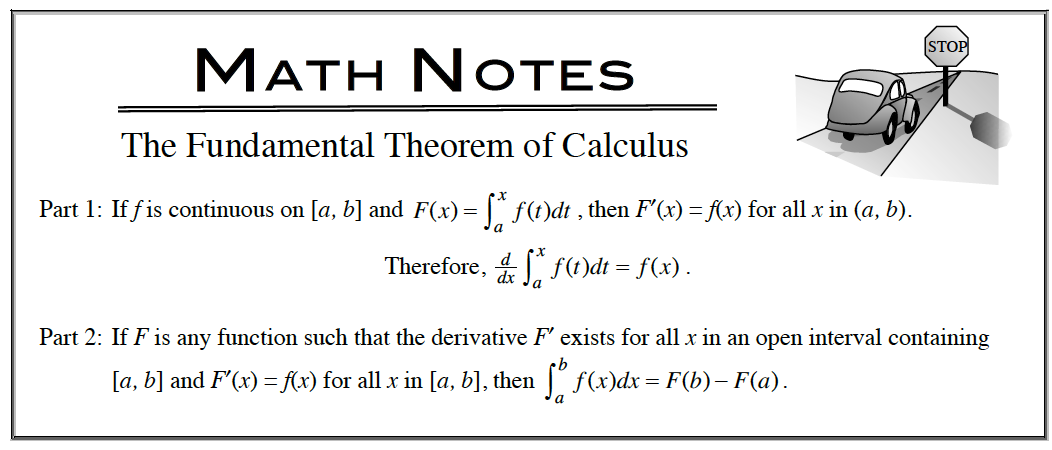### Home > APCALC > Chapter 5 > Lesson 5.3.3 > Problem5-137

5-137.

Multiple Choice: $\frac { d } { d x } \int _ { 0 } ^ { \pi / 3 } \operatorname { sin } ( x ) d x =$ Homework Help ✎

1. $\frac { \sqrt { 3 } } { 2 }$

2. $\frac { 1 } { 2 }$

3. $0$

4. $- \frac { 1 } { 2 }$

5. undefinedNotice that the Fundamental Theorem of Calculus, part 1 states that the derivative of an INDEFINITE integral is the original function.
This, however, is a DEFINITE integral, and a definite integral has a finite sum. What is the derivative of a finite value?